## Understanding Fractions (Grades 5 to 8)

### Regina Catholic Schools Regina, Saskatchewan

One of the areas most frustrating for teachers and students alike is the study of fractions, specifically operations with fractions. Year after year, students learn and forget how to add, subtract, multiply and divide with fractions. The main reason students have difficulties with fractions is that they seem to want to memorize formulas or algorithms instead of understanding them.

Manipulatives, when used to introduce concepts about fractions, help students understand the ideas about fractions. Pattern blocks and fraction blocks have many uses in learning mathematical concepts, but they are especially useful in learning about fractions.

For those unfamiliar with pattern blocks, the basic set consists of 6 shapes:

 the yellow hexagon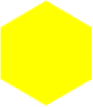the blue rhombus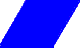the orange square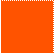the green trianglethe red trapezoid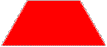and the beige rhombus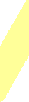In recent years, fraction blocks have become available to supplement the basic pattern blocks. The additional shapes are:

the pink double hexagonand the black chevron.For the purpose of studying fractions, we will be using the double hexagon, the hexagon, the chevron, the trapezoid, the blue rhombus and the triangle.

Have students work in pairs with the blocks. Have the students discover the relationships between these 6 blocks:

• how many green blocks are equivalent to a blue block, or a red block, or a black block, or a yellow block, or a pink block?
• how many red blocks are equivalent to a yellow block, or a pink block?
• how many blue blocks are equivalent to a black block, or a yellow block, or a pink block?
• How many black blocks are equivalent to a pink block?

We will use the pink double hexagon as the unit 1.Each student should have an outline of this form to use with the blocks. The teacher should have this outline on an overhead to work along with the students.

Lesson 1-Equivalent Fractions

Have the students cover the form with yellow blocks. What fraction of the whole does 1 yellow block represent? (1/2) Write this fraction down on the overhead, the blackboard or chart paper for future reference.

Clear the outline. Have them cover the outline with black blocks. What fraction of the whole does 1 black block represent? (1/3) 2 black blocks? (2/3) 3 black blocks? 3/3 Write these fractions down.

Clear the outline. Have them cover the outline with red blocks. What fraction of the whole does 1 red block represent? (1/4) 2 red blocks? (2/4) 3 red blocks? (3/4) 4 blocks (4/4) Write these fractions down.

Which of these 4 fractions is the same as 1/2? Ask students to show this on their outline. Ask a student to show it on the overhead.

Clear the outline. Have the students cover the outline with blue blocks. What fraction of the whole does 1 blue block represent? (1/6) 2 blue blocks? (2/6) 3 blue blocks? (3/6) 4 blue blocks? (4/6) 5 blue blocks? (5/6) 6 blue blocks? (6/6) Write these fractions down.

Which of these fractions is the same as 1/2? Ask students to show and explain this using their blocks.

Which of these fractions is the same as 1/3? 2/3? Ask students to show and explain this using their blocks.

Clear the outline. Have the students cover the outline with green blocks. What fraction of the whole does 1 green block represent? (1/12) ......... 12 green blocks? (12/12) Write these fractions down.

Have the students show and list all fractions equivalent to 1/2.

Have the students show and list all fractions equivalent to 1/4, 3/4, 1/3, 2/3, 1, etc.

Have students list all fractions smaller (but greater than 0) than 1/2.

Have students list all fractions greater (but smaller than 1) than 1/2.

Have students list from smallest to greatest all the fractions which can be represented by the blocks (up to 1).

If the outline represents 1, show 1 1/2 using yellow blocks, or red blocks.

Show 2 1/4 using a variety of blocks.

This lesson allows students to understand and become familiar with equivalent fractions and the order of fractions.

Have students cover their outline with a yellow block and a red block. Show this at the same time on the overhead.

What fraction of the whole does this represent? Notice that the word "addition" has not been used yet. Some students may respond that we can't tell because the blocks are different. Ask students if they can "replace" blocks so that all the blocks are the same. The yellow block will then be replaced by 2 red blocks. Connecting with what they learned in the previous lesson, they should be able to answer the question (3/4).

At this point, the teacher may wish to write the following on the board or chart paper:

1/2 + 1/4 = 3/4 and show with the blocks that this represents what they have just done.

Let's try another one: cover the outline with a red and a blue. What fraction of the whole does this represent?

Generally speaking:

• ask what fraction of the whole it represents;
• exchange the blocks with "equivalents".

As students become more proficient, start off by writing the equation on the board and ask which blocks are needed to represent this equation: 2/3 + 1/6 = ?

The blocks needed to start would be 2 blues and 1 green. Students can then make the exchange and find the answer (5/6).

You may discover that your students become very good at visually "seeing" the answer. Later, some of these students may be able to "visualize" fractions and this will be a great asset for them.

Practice many additions, making sure the manipulatives and the symbols are used. Do not proceed to symbols only until students are ready. Even when students have moved to the symbolic addition of fractions, always allow them to use the manipulatives if needed; often it is only to refresh their memory or test out some new idea.

Make sure you proceed to fractions greater than 1 and 2 as the students become better. You may even ask students to make up their own equations and show them on the overhead for others to solve or see how they found the solution.

Lesson 2 should last for a few lessons. Time spent on understanding fractions is time well spent.

Lesson 3-Subtraction of Fractions

When students are comfortable with addition, they can then proceed with subtraction.

The principle is the same as for addition - the idea of equivalent fractions.

Lesson 4-Multiplication of Fractions

Cover the outline with 1 yellow block. Tell students you only want 1/2 of that block. How can they show that? (They could replace the yellow block with 2 red blocks and take one of these away). Now,what fraction of the whole outline does this block represent? (1/4)

Next write the following equation on the board: 1/2 x 1/2 = 1/4 and show with the blocks that this is what was just done.

Repeat using a yellow block but wanting 1/3 of the block. (The yellow block will be replaced with 3 blue blocks and one of them will be kept). What fraction of the whole outline does this block represent? (1/6)

Write the equation on the board (1/2 x 1/3 = 1/6) and show this with the blocks. Continue with (1/3 x 1/2 = 1/6).

Next write a new equation on the board (1/4 x 1/3 = ) and ask students to show and explain how they can solve this using the blocks.

Keep the equations on the board. Some students may discover that there is a pattern when it comes to multiplying fractions (multiplying the numerators and multiplying the denominators).

Many lessons can be used to develop these concepts, eventually using fractions with numerators larger than 1 and using fractions greater than 1.

Don't forget to always show the link between the blocks and the symbols.

Lesson 5- Division of Fractions

Yes! Pattern blocks can be used to show the concept of division of fractions.

Write the following equation on the board: 1/2 / 1/2 = . Indicate that this is not the same as 1/2 x 1/2. Cover the outline with 1 yellow block representing the first 1/2. Next pick up a second yellow block representing the second 1/2 and ask the following question: How many of this block would fit in the block on the outline? (1) Write the answer at the end of the equation. The question represents this particular concept of fraction.

Try another 1/2 / 1/4 = . Cover the outline with 1 yellow block to represent 1/2 and pick up a red block to represent 1/4. How many of this red block does it take to cover the yellow block? (2). Write the answer at the end of the equation.

Try 4 or 5 more. Students will become very good at this. Keep writing the equations and the answers on the board. The pattern is not so obvious but students may realize that by inverting the second fraction, they can find the answer.

What is important here is that students understand what it means when we divide fractions. It will help them visualize the kind of answer they should be getting, to estimate the answer. This is a very valuable tool.

Notes

 At any time during the lessons, please allow time for students to express their thinking and concerns about what they are learning. All of these "lessons" require more than one math period. The outline can be made by tracing around one pink  piece and photocopying it for the students and making an overhead for the teacher. Pattern blocks are sold in buckets. Three buckets are the minimum number of buckets a classroom should have to ensure that students have enough of the pieces needed. Fraction blocks (the 2 new shapes) are sold separately. Blocks can be stored in Ziploc type bags, one bag for each pair of students (this saves a lot of class time). Pattern blocks and fraction blocks can be purchased through Exclusive Educational Products (www.exclusiveeducational.ca), Spectrum (www.spectrumed.com) and other places. Also available are pattern blocks for use on the overhead projector, magnetic fraction blocks (containing all the shapes needed for fractions) and binders of activities related to pattern blocks (French and English); these activities relate to problem solving, geometry, perimeter, area, fractions, etc. These binders include activities for K-8. In particular the binder called Fraction Blocks (French and English) is devoted exclusively to fractions. These binders are available through Exclusive Educational Products (www.exclusiveeducational.ca). The use of the pattern blocks and fraction blocks to teach understanding of concepts related to fractions limits the fractions which can be used. But this is not necessarily a disadvantage. If students understand the concepts using these fractions, they will be able to make the connections to other fractions.

According to the Saskatchewan Mathematics Curriculum:

 students should be able to add and subtract fractions with like denominators using manipulatives and pictures in grades 5 and 6; students should be able to add and subtract fractions with unlike denominators using denominators using manipulatives and pictures in grades 7 and 8; students should be able to multiply fractions using manipulatives and pictures in grades 7 and 8; students should be able to divide fractions using manipulatives and pictures in grades 7 and 8.

Only in grades 8 and 9 will students be expected to divide fractions using symbols only.

Student Evaluation

You can even evaluate students' knowledge of fractions using manipulatives.

As a test, hand out a sheet with problems involving fractions and let the students use the pattern blocks. Have the students' draw the outline of the blocks they have used to solve the problems.

What you will be assessing is the students' understanding of the concepts involved with fractions and operations.

Other ways of evaluating students' understanding using blocks can be devised. You may wish to share some of your ideas about teaching and assessing by sending them to this web site. Don't be shy!

Experiment and have fun with manipulatives and fractions.

Persevere and watch the reactions of your students.